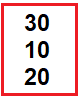# Scoping Rule in C++

## Scoping Rule in C++ with Examples:

In this article, I am going to discuss Scoping Rule in C++ Language with examples. Please read our previous article, where we discussed Static Variables in C++ with examples.

##### Scoping Rule in C++:

Let us understand the levels of scopes that C++ supports with an example.

int x = 10;
int main(){
int x = 20;
{
int x = 30;
cout << x << endl;
}
cout << x << endl;
}

Here we have 3 ‘x’ variables. One is a global variable and one is local to the main function and one is local within a block. So, C++ has block-level scope. Now inside this block. If we write ‘cout << x’ then which value will be printed? It will print the nearest value of ‘x’ which is 30. And outside the block, if we say ‘cout << x’ then which ‘x’ will be printed? It will print the value that is its nearest scope which is 20.

So, if we run this program, we will get the value 30 and then 20 and inside the main function, the ‘x’ with the value ‘10’ will never be accessible inside the main function. Whenever we say ‘x’ this will be accessing this local variable ‘x’ of value 20 but if we want to access the global variable ‘x’ of value 10 then we should write ‘cout<<::x<< endl’. So, that’ll be accessing that global ‘x’. So, C++ has block levels scope. Now let us look at the complete program.

##### Scoping Rule Program in C++:
```#include <iostream>
using namespace std;
int x = 10;
int main ()
{
int x = 20;
{
int x = 30;
cout << x << endl;
cout << ::x << endl;
}
cout << x << endl;
}
```
###### Output: